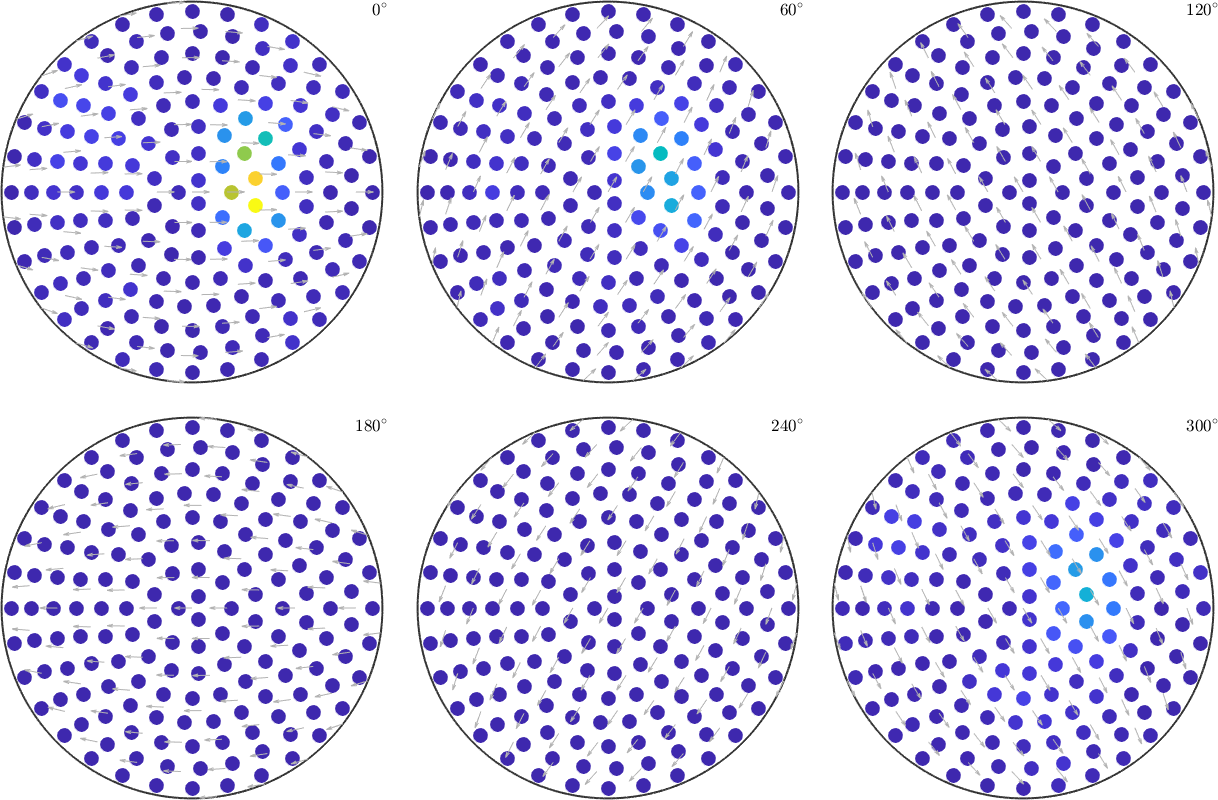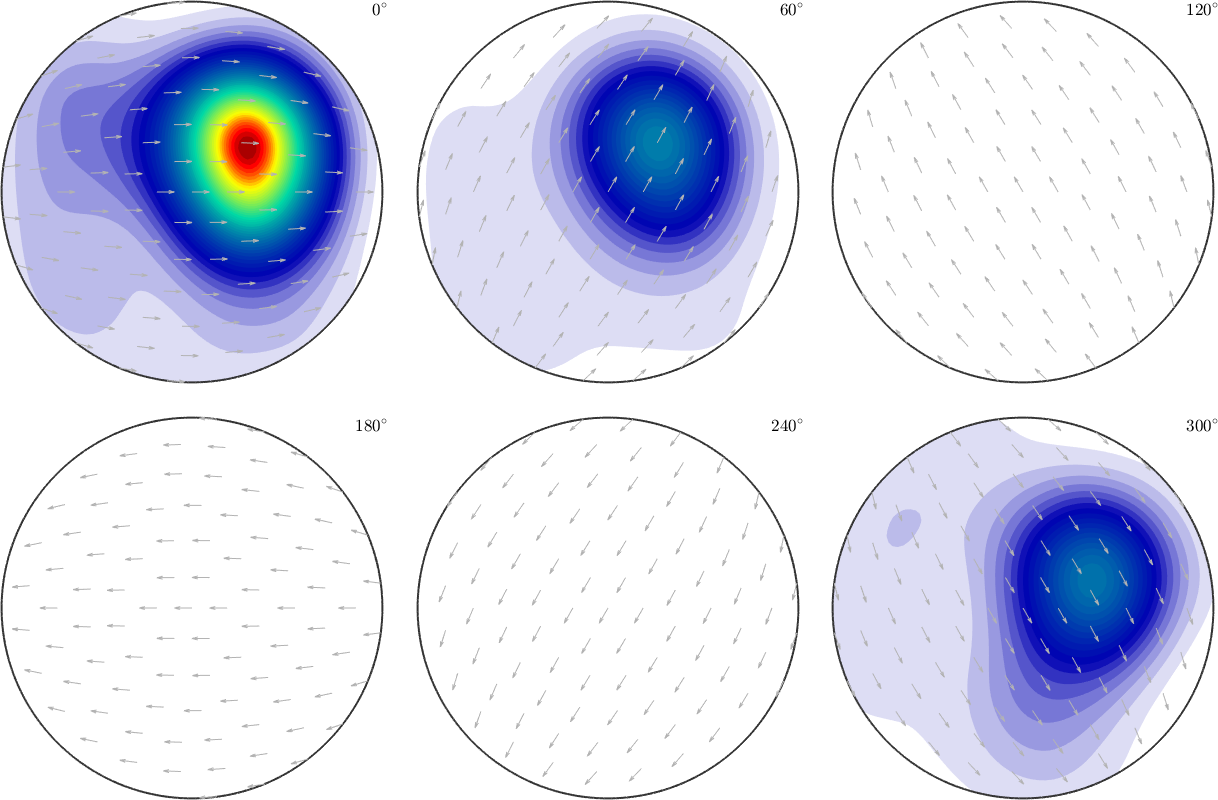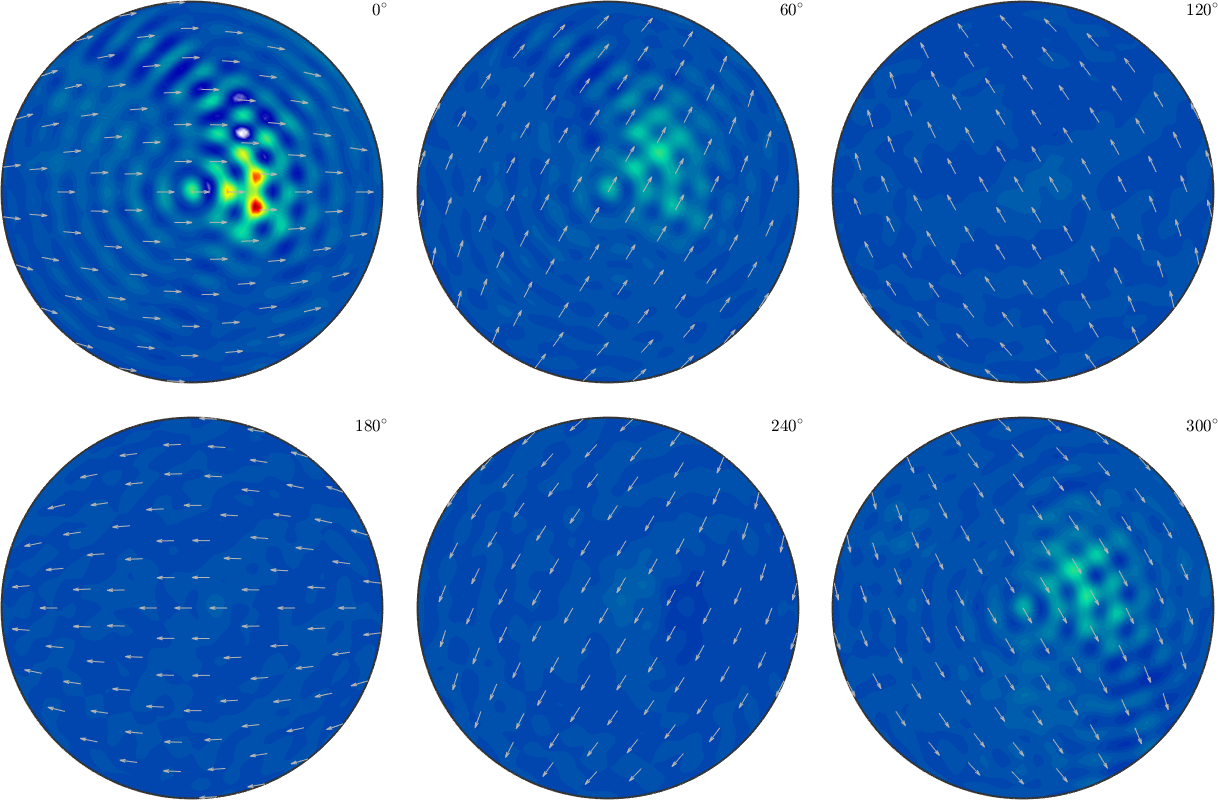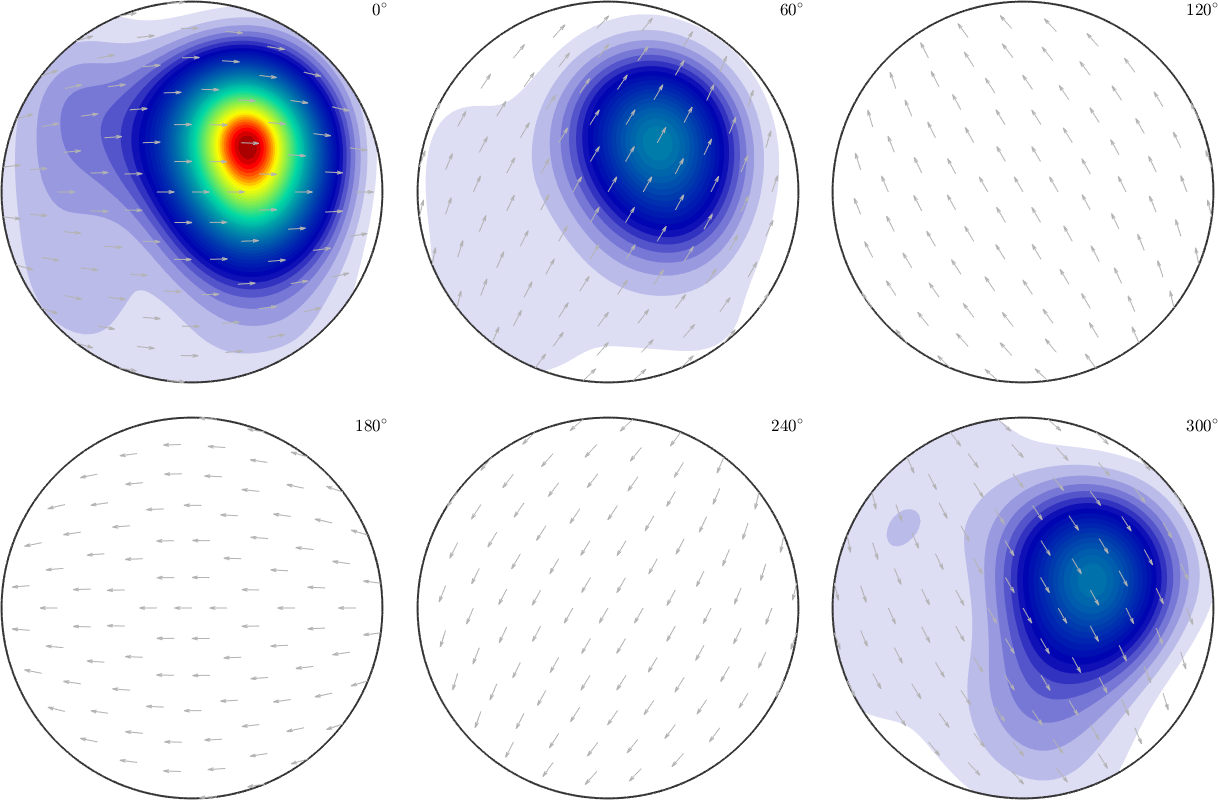Rotational Approximation and Interpolation edit page

On this page, we want to cover the topic of function approximation from discrete values on the Rotation group. To simulate this, we have stored some nodes and corresponding function values which we can load. The csv-file contains the Euler angles $$\phi_1$$, $$\Phi$$ and $$\phi_2$$ of the nodes and the function value in the fourth column. Lets import these data using the function load

The second output S is a struct that contains a field S.values with the function values from the fourth column. Next, we can make a section plot to see, what we are dealing withNow, we want to find a function which coincides with the given function values in the nodes reasonably well.

## Interpolation

Interpolation is done by the interpolate command of class SO3FunThe interpolation is done by lsqr. Hence the error is not in machine precision.

If we don't restrict ourselfs to the given function values in the nodes, we have more freedom, which can be seen in the case of approximation.

## Approximation

In contrast to interpolation we are now not restricted to the function values in the nodes but still want to keep the error reasonably small.

One way is to interpolate the function similary as before, without the option 'exact'.

Another way is to approximate the rotational function with a series of Wigner-D functions (Harmonic series). We don't take as many Wigner-D functions as there are nodes, such that we are in the overdetermined case. In that way we don't have a chance of getting the error in the nodes zero but hope for a smoother approximation. This can be achieved by the approximation command of the class SO3FunHarmonicPlotting this function, we can immidiately see, that we have a much smoother function. But one has to keep in mind that the error in the data nodes is not zero as in the case of interpolation.

But this may not be of great importance like in the case of function approximation from noisy function values, where we don't know the exact function values anyways.

The strategy underlying the approximation-command to obtain such an approximation works via Wigner-D functions (Basics of rotational harmonics). For that, we seek for so-called Fourier-coefficients $${\bf \hat f} = (\hat f^{0,0}_0,\dots,\hat f^{N,N}_N)^T$$ such that

$g(x) = \sum_{n=0}^N\sum_{k,l = -n}^n \hat f_n^{k,l} D_n^{k,l}(x)$

approximates our function. A basic strategy to achieve this is through least squares, where we minimize the functional

$\sum_{m=1}^M|f(x_m)-g(x_m)|^2$

for the data nodes $$x_m$$, $$m=1,\dots,M$$, $$f(x_m)$$ the target function values and $$g(x_m)$$ our approximation evaluated in the given data nodes.

This can be done by the lsqr function of Matlab, which efficiently seeks for roots of the derivative of the given functional (also known as normal equation). In the process we compute the matrix-vector product with the Fourier-matrix multible times, where the Fourier-matrix is given by

$F = [D_n^{k,l}(x_m)]_{m = 1,\dots,M;~n = 0,\dots,N,\,k,l = -n,\dots,n}.$

This matrix-vector product can be computed efficiently with the use of the nonequispaced SO(3) Fourier transform NSOFT or faster by the combination of an Wigner-transform together with a NFFT.

We end up with the Fourier-coefficients of our approximation $$g$$, which describe our approximation.

Assume we have some experiment which yields an ODF or some general SO3Fun, i.e. some evaluation routine.

Now we want to compute the corresponding SO3FunHarmonic. If our odf is an SO3Fun or @function_handle we can directly use the command SO3FunHarmonic.

If there is an physical experiment which yields the function values for given orientations, we can also do the quadrature manually.

Therefore we have to evaluate on an specific grid and afterwards we compute the Fourier coefficients by the command SO3FunHarmonic.quadrature.

Lets take a look on the resultFurthermore, if the evaluation step is very expansive it might be a good idea to use the smaller Gauss-Legendre quadrature grid. The Gauss-Legendre quadrature lattice has half as many points as the default Clenshaw-Curtis quadrature lattice. But the quadrature method is much more time consuming.